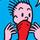## Social Question# Is this a good math trick?

Asked by LostInParadise (29005) February 3rd, 2018

Write a 9 digit number such that the seventh and ninth digits (1’s and 100’s columns) are 1 and 9 in either order.

Form a second 9 digit number by transposing the first and third digits, fourth and sixth digits, and seventh and ninth digits.

Using a calculator, subtract the smaller number from the larger one. The number showing will be exactly divisible by 792, which can be verified by doing the division and ending up with a whole number.

Observing members: 0Composing members: 0Too early in the morning for math problems. I’ll try again later.

seawulf575 (12923)“Great Answer” (0) Flag as…